## Thursday, December 27, 2018

### The advantages and disadvantages of using a Halbach array with a BLDC (PMSM) motor

In the last post it was shown that the length of the rotor magnets has an impact on the specific torque density of an electric motor. Longer magnets will, in general, produce a larger flux density at the poles but this comes at the expense of a larger flux gap. Therefore, the optimum magnet length for our motor model was around 2-4 mm.

However, there is an alternative arrangement of magnets in the rotor that, according to many a forum post all over the internet, will significantly increase the torque density of any motor.

## The Halbach array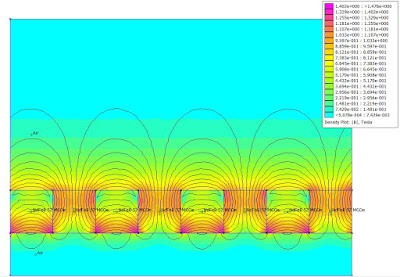Credit: Wikipedia
If you are not familiar with the concept of a Halbach array then its wiki page has all the relevant information. In this post we will be testing the impact of adding a simple Halbach array to our model motor in FEMM.

## Four different scenarios for a simple motor model

While more details regarding this motor can be found in this post a brief description is as follows: It is a 6 slot, 8 pole motor with three phases wound as concentrated windings in a pattern of ABCABC. All current in the windings is on the q-axis and in this case there is a 4.5 mm flux gap with 4 mm long magnets. FEMM simulation results shown below.

Back iron only
Torque output: 0.714 N.m

Back iron and Halbach
Torque output: 0.714 N.m

Halbach Only
Torque output: 0.683 N.m

Neither back iron or Halbach
Torque output: 0.435 N.m

It's clear that adding a Halbach style arrangement of magnets to the rotor has no impact on the torque produced when back iron is also used. However, it makes a considerable difference when the rotor back iron is removed, giving roughly 50% more torque than the non-Halbach arrangement.

## A simplified example

The reason for this is clear when you look at a simplified arrangement of magnets.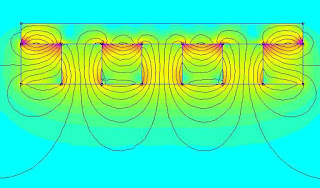Back iron and Halbach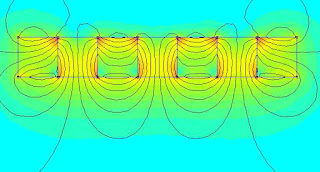Halbach OnlyNeither back iron or Halbach

The back iron produces a high magnetic permeability path for the permanent magnet 'flux' to pass through. The use of a Halbach array eliminates the need for back iron and so the use of both a Halbach array and back iron will only increase the cost and weight of a motor, with no improvement in performance.

If we draw a downwards line from the surface of the exposed magnets in the images above and plot the flux density at each point we see the following.

It is clear that the flux density is the same with or without the Halbach configuration provided that back iron is used. Therefore, a Halbach array does not act to redirect the flux from one side of a magnet, concentrating it on the other, as is sometimes stated. Therefore, it only makes sense to use a Halbach array when designing a motor which has no 'back iron' in the rotor. However, having no back iron in the rotor is essentially the same as having an infinite flux gap. As was shown in the last post, the highest torque density was achieved for our model motor when the flux gap was kept small using thin magnets. So not only would a Halbach configuration for this motor be considerably more expensive to manufacture, it would also have a lower torque density.

There are of course exceptions to this example. Completely core-less electric motors (no stator or rotor iron) such as this example often use Halbach arrays as a means to increase their otherwise terrible torque density. The benefit to this design is that the lack of iron core losses means that these motors can be quite efficient provided eddy currents in the windings and magnets is adequately controlled. They also produce no cogging torque. As far as I can tell, core-less motors are also popular among hobbyist because they eliminate the need to cut your own Fe-Si steel lamination. If you are looking to make a one off custom motor as a hobbyist my advice would be to re-use an off the shelf stator (either new or from a donor motor) and modify it to your own needs. This approach will always give you a higher torque density than a core-less motor and will often be cheaper since you don't need to purchase as many, or as large, expensive magnets or litz wire for the windings.

### Understanding BLDC (PMSM) electric motor constants - Optimal magnet length for high torque density

In the last post it was shown that the torque density of a motor can be improved by making the flux gap as small as possible. It was also seen that the rotor magnets are considered part of the flux gap. Therefore, it would appear that an ideal motor will always have rotor magnets that are as thin as possible.

However, it is also well known that the longer you make a permanent magnet, the larger the flux density at its surface. This raises an important question: Are long magnets and a large flux gap better than short magnets and a small flux gap when it comes to producing the most torque?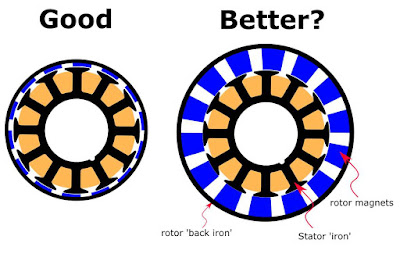Small flux gap and magnets on the left, large magnets and flux gap on the right.

## The short answer:

For a 'hobby grade' out-runner electric motor the optimal magnet length will depend on your exact motor design, but in general, it will likely be around 1 to 4mm in length. Shorter magnets see a rapid fall off in their flux contribution to the airgap while longer magnets increase the magnetic reluctance of the magnetic circuit, reducing the stator contribution. Very long magnets will also cause the stator and rotor back iron to saturate which reduces performance.

Read on if you would like a more detailed understanding.

## Permanent magnet self demagnetisation

Below are four magnets  modelled in FEMM with a length of 1, 2, 4 and 8 mm

Despite each magnet being made of the same material there is a clear difference in the flux density present at the surface poles. The reason for this effect is that shorter magnets have a higher demagnetisation factor in that direction. The demagnetisation factor reduces the B field inside the magnet and is dependent upon the magnet geometry. The concept of a demagnetisation factor also applies to soft magnetic materials, not just permanent magnets. Long magnets will have a lower demagnetisation factor than shorter magnets. Unfortunately, there is no simple equation that can be used to describe the demagnetisation factor for something as basic as a cube. However, there is a simple relationship for an ellipsoid. Note that if you have a magnetic circuit that makes a closed circuit then the demagnetisation factor is zero but there are also no magnetic poles.

If we draw a line projecting out from the surface pole of each magnet and measure the flux density at each point we get the following plot.

Here we can see that the flux density in air for the 8 x 8 mm magnet at a distance of ~ 10 mm is the same as the surface (0 mm distance) for the 1 x 8 mm. This trend of increasing flux density with magnet length does not continue forever. The flux density in air at a distance of 0.5 mm from the surface of the magnets is plotted vs magnet length below.

As the magnet is made longer, and its demagnetisation factor in that direction decreases, the field produced at the surface poles would eventually approach that of the magnets remanent magnetisation. The plot above will look quite different if the same magnet was instead placed into the rotor of a motor since you then also have high magnetic permeability material in the stator and rotor helping guide the flux from the magnet, reducing its demagnetisation factor. However the overall concept remains the same.

## Effect of magnet length on the torque produced by a simple motor

As in the previous post, we can use a simple model of a motor to test how different magnet lengths impact the torque produced for a fixed winding current. Below are four different scenarios. Each motor has a gap between the stator and the magnets of 0.5 mm. Therefore, the total flux gap is given by the magnet length + 0.5 mm.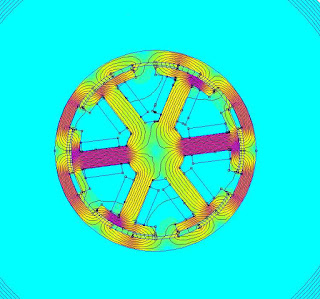1 mm rotor magnet2 mm rotor magnet4 mm rotor magnet8 mm rotor magnet
We can see a few things right away. First, the flux density in the rotor 'back iron' increases considerably as you make the magnets longer to the point that the back iron begins to saturate. This can also be seen in the stator teeth. Secondly, we can see that more flux escapes the rotor and fringes into the surrounding air. This is due to the saturation of the back iron.

The flux density in the flux gap is plotted below with different length magnets. The flux contribution from only the stator windings was estimated by removing the magnets and simulating the motor. The same was done for the flux density contribution for the magnets, this time with the stator winding current set to zero.

It is clear that as you make the magnets longer the flux contribution from the stator becomes smaller due to the increase in the flux gap size. On the other hand, the flux density contribution from the magnets increases as they are made longer. Based on this plot we would expect that the torque will continue to rise as the magnets are increased in length. However, when the rotor torque is plotted with respect to the magnet length we can see that maximum torque is reached for magnets that are about 4 mm long. Further increasing the magnet length sees the torque slowly fall off.

This fall off in torque for magnets longer than about 4 mm is likely due to the stator core and rotor back iron beginning to saturate. Perhaps more interestingly is if we plot the specific torque density (torque per unit volume) and gravimetric torque density (torque per unit mass).

When the magnets are made longer they are adding mass and volume to the entire motor while the torque gradually decreases. Therefore, there is a sharp fall off in the specific torque density for magnets longer than about 2 mm. Note that in the above example only the magnet length was changed. If more than one parameter was refined for, such as the thickness of the back iron, then the results will differ from those above.

In addition to just the torque output there are many other factors which need to be considered when you change the length of the magnets contained in a motor. A few that come to mind may include:
• Core losses are likely to increase when magnet length is increased as the stator and rotor iron is operating closer to saturation. Stray flux from the magnets may also cause more eddy current losses in the windings.
• Magnets are easily the most expensive part of a hobby grade electric motor. Therefore, motor cost will increase considerably if you were to use longer magnets, even if a redesigned motor did see a slight increase in torque with magnet length.
• Increasing the magnet length will add more mass to the rotor which increases its moment of inertia, reducing the dynamic response time of the motor.
• Cogging torque generated by the salient poles of the stator will likely be much worse with more powerful magnets
• There are many different grades of magnetic materiel. Using more or less powerful magnets would likely change the optimum magnet length.

## Conclusion

Increasing the length of the permanent magnets used in a 'BLDC' (PMSM) motor will increase the torque produced only up to a point. The optimum magnet length will depend on many factors, but as a general rule of thumb, this length will be between 1 and 4 mm for 'hobby grade' out-runner electric motors constructed with back iron in the rotor. Further increasing the magnet length will only reduce the motor performance and increase the motors production costs.

If you have noticed any errors in the above article then please let me know. If you would like to play around with any of the models shown in this post in FEMM you can find the files hosted hereThis tutorial gives you enough information to get started if you have never used FEMM before.

## Wednesday, December 26, 2018

### Understanding BLDC (PMSM) electric motor constants - Optimal flux gap for high torque density

For many weight sensitive applications in robotics, it is desirable to have high torque density actuators. It is also often desirable to have relatively low gear ratios as this improves un-sensored output torque accuracy and helps to minimise rotational inertia, which improves angular acceleration. This is the basis of Ben Katz low-cost modular actuator design. There is, therefore, a need for electric motors with as high a gravimetric torque density (torque per unit mass) as possible.

Despite this, I have so far been unable to find much information online in the 'hobbyist community' (i.e. non-academic) about which aspects of an electric motor are important for torque density and how they can be optimised. Therefore, for the next series of posts I will be using FEMM and a simple motor model to try and develop a working understanding of how different motor parameters (e.g. flux gap size, magnet length, stator tooth shape, slot and pole number etc.) impact the torque density of a brushless permanent magnet synchronous motor.

Let's get started.

## The flux gap

The flux gap is the distance between the high magnetic permeability material in the stator (stator 'iron') and the corresponding high magnetic permeability material in the rotor (rotor 'back iron'). This material is normally made of thin laminations of Fe-Si steel.

It is well known that, in general, the flux gap should be as small as possible.

It is important to note here that the flux gap includes the magnets. Rare earth magnets (magnetised or un-magnetised) have a magnetic permeability essentially the same as air. Therefore, from the stators perspective, a magnet is no different than air and should be included as part of the flux gap.

Let's start by considering two simple magnetic circuits simulated in FEMM; one with a 1 mm flux gap and one with a 4 mm flux gap.1 mm flux gap core4 mm flux gap
The magnetic circuit consists of a ring of soft iron (literally pure annealed iron), copper windings represented by the green rectangles (2A, 250 turns) and an air gap in the ring. The flux density (unit of Tesla) is represented by how close the lines of flux are together and by the colour, with red being the highest density and blue being the lowest. The flux density in the ring with the small flux gap is clearly the largest. This ring also has the least amount of flux 'leaking' out into the surrounding air.

The reason for this difference is that an air gap increases the magnetic reluctance of the circuit. Magnetic reluctance is to flux in a magnetic circuit what resistance is to a current in an electric circuit. Therefore, the magnetic flux in the circuit is dependent upon the total magnetic reluctance and the applied magneto-motive force (turns times current) just as an electrical current is dependent upon the total resistance in an electric circuit and the applied voltage. For a nice overview of the concept check out Ben Krasnow's video on the topic.

Let's look more closely at how the flux changes over the flux gap itself. We can do this by drawing a line over the flux gap and measuring the flux density at each point on the line.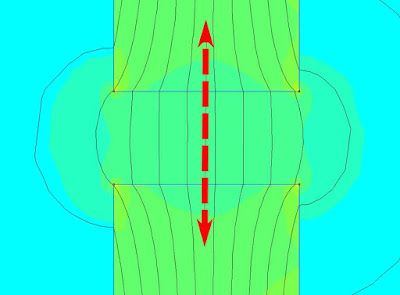The 4 mm flux gap and line which we will measure flux density
Doing this for both the 1 mm and 4 mm flux gap it is clear that the flux in the middle of each gap remains constant. It can also be seen that the flux is four times smaller in the 4 mm flux gap than the 1 mm flux gap. Therefore, in order to produce the same flux density in the 4 mm gap, we would need to either add four times as many windings at the same current or alternatively, keep the same number of windings and add four times as much current. This concept can also be applied to electric motors and explains why engineers generally do everything they can to keep the flux gap as small as possible.

The flux density in the flux gap can be approximated using the following equation:

B=\frac{\mu_{0}NI}{g}

where B is the flux density (Tesla), mu_{0} is the magnetic permeability of free space (4\pi\times10^{-7}), N is the number of turns of wire, I is the current (Ampere) and g is the flux gap (meters). Plotting B vs g we see the following:

This equation assumes that the reluctance of the iron core is negligible. This is a safe assumption in this example as reluctance of the Fe core is around 10^{-7} times smaller than the reluctance of the flux gap and so can be disregarded. However, if your core is close to saturation, as would be the case if you reduce the flux gap to zero, then this will not always be the case. Also, this equation can only be used for a constant cross-section like that of the 'racetrack' core shown above, but does provide a good starting point for cores of other shapes. For best results a FEA package like FEMM (its free!) will give the best approximation.

Unlike the simple magnetic circuits shown above, the flux gap problem is made more complicated for BLDC motors for a few reasons:
1. There are multiple flux gaps. The stator flux must travel across to the rotor and back again and can do so at multiple points.
2. The magnetic permeability, and hence the magnetic reluctance, of the ferromagnetic stator and rotor back iron, is not constant but instead depends on the total amount of flux in that region.
Note that simply embedding the magnets in the rotor back iron does not eliminate the flux gap, it only moves it further back into the rotor. Embedded rotor magnets do have their own advantages (improved field weakening performance, control over reluctance torque) but they are topics for another day.

## Effect of flux gap size on torque for a simple motor

In order to explore the impact of the flux gap size on something more closely resembling a real  motor I have simulated a 6 slot, 8 pole 'out runner'. The motor was sketched in F360 and exported as a dxf file for use in FEMM. It has a stator diameter of 57 mm and a rotor length (into screen) of 10 mm. This motor has three phases which are wound with concentrated windings (double layered) as ABCABC. A current of 50A is supplied on phase A, and -25A is supplied on phase B and C so that all of the flux is directed on the Q-axis where it will produce the most torque.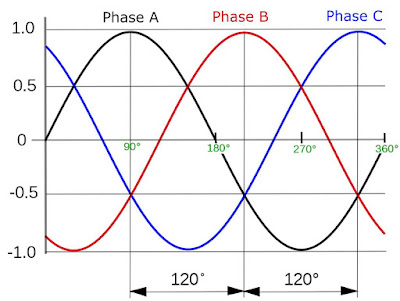The motor is simulated with a fixed current at 90 degree
Shane Colton's blog post on field orientation has a good rundown on the q-axis and d-axis argument.  In short, the phase with the most current on it (phase A) is 90 electrical degrees ahead (q-axis) of the direct flux of the magnets (d-axis) where it will produce the most torque per amp. If the rotor was rotating then so too would the magnetic field generated in the stator so that the torque remains constant and proportional to the current.Flux density due to the stator windings only (magnets removed)
However, in this simulation, both the current and rotor position are fixed and we are instead only solving for the flux density generated in the air gap by the stator windings and the magnets.
In the simulation seen below the flux is seen to be concentrated in the high magnetic permeability stator teeth and in the back iron of the rotor. This flux almost exclusively crosses at the flux gap.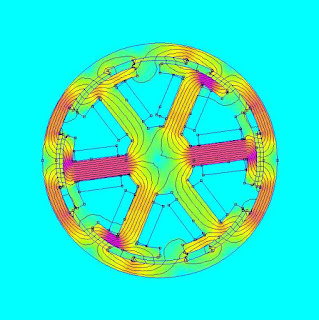1 mm flux gap
For comparison, a motor with a 3mm flux gap is shown below. Here we can see that the total flux density in the stator teeth and back iron is greatly reduced due to the increased magnetic reluctance in the magnetic circuit. This decrease is also seen in the flux gap where the magnets are located.3 mm flux gap
If you would like to play around with these models in FEMM you can find the files hosted here. This tutorial gives you enough information to get started if you have never used FEMM before.

Using FEMM it is also possible to estimate the static torque that this 10 mm long rotor would produce. The torque and flux density within the flux gap with respect to the flux gap size is shown below.

We can see two things quite clearly: i) the torque produced by the motor is dependent upon the flux density in the air gap and ii) the torque falls off asymptotically as the flux gap size is increased. Note that in this example we are increasing the diameter of the rotor to increase the flux gap. Since motor torque increases with the square of the flux gap diameter the fall off in torque would be much steeper if we were to instead decrease the size of the stator to increase the flux gap.

From the above discussion it is clear that, in general, we want to have as small a flux gap as physically possible so as to increase the motors torque output and, therefore, it's motor constant. However, aside from needing to consider manufacturing tolerances, we also need to consider the thickness of the magnets. In general, if you make the rotor magnets longer then the flux density at their poles is also increased. This will act to increase the torque output of your motor.

The impact of magnet length to motor torque will, therefore, be the topic of the next post.

## Conclusion

Increasing the size of the flux gap for a motor will increase the magnetic reluctance in the magnetic circuit which reduces the flux density in the air gap. The torque generated by a motor is proportional to the flux in the air gap. Therefore, increasing the size of the flux gap will reduce the torque generated by a motor for a fixed winding current, which reduces the overall motor constant.

Equations were produced in this post with the help of arachnoid.com and are based on those found in the book Electric Motors and Drives: Fundamentals, types and applications by Austin Hughes. If you have noticed any errors in the above article then please let me know.

## Tuesday, December 25, 2018

### How to estimate the torque of a BLDC (PMSM) electric motor using only its Kv and current draw

There exists a fundamental relationship between an electric motors velocity constant (K_{V}), armature* current (I_{A}) and torque (\tau)This relationship is as follows:

\tau \approx  \frac{8.3 \times I_{A} }{K_{V}}

were \tau is in N.m, I_{A} is in A and  K_{V} is in RPM/V. This relationship is extremely useful since most 'hobby grade' BLDC/PMSM manufacturers do not publish the usual motor constants you would expect from an industrial product while K_{V} and peak I_{A} is normally specified on sites like hobbyking.com.

The above relationship works for two reasons:
1.  A motors K_{V} and its torque constant (K_{\tau}) are fundamentally the same thing.
2.  The torque generated by a motor for a given current is governed by K_{\tau}.
Of course, there are limitations to this approach. If a motor is close to saturation or if the current waveform supplied by a motor controller does not exactly match a motors back EMF waveform (i.e. the current is not exactly on the q-axis at all times) then your torque will be less than that suggested above. However, my own testing shows that this approximation is quite accurate when a 'hobby grade' PMSM motor is driven using field oriented control (FOC). Even when not using FOC or a PMSM this approach should still provide a good starting point.

Read on if you are interested in a more detailed understanding of why this relationship works and the limitations of this approach.

## Fundamental torque production

Electric motors do useful work by producing torque and rotation. The amount of steady state torque produced by a well optimised and non-salient machine** with a specific volume (specific torque density) is ultimately dependent upon three factors:
• The average flux density acting upon the armature. For a BLDC/PMSM motor this 'flux' is provided by the rotor's permanent magnets.
• The average current that can be maintained by the armature without overheating**. For a BLDC/PMSM motor the armature consists of the copper windings in the stator.
• The total length of the armature windings which has 'flux' acting upon it. For a BLDC/PMSM motor this length represents the number of turns of wire in the stator armature which interact with the 'flux' provided by the rotor.
In other words, all the complexity of an electric motor ultimately boils down to the "BIL" Lorentz force law:

Force = Magnetic field \timesCurrent\times Conductor length

Increase one of the above terms without negatively impacting another and you have increased the torque output of your system. When discussing motor constants I find it useful to keep this simple relationship in mind.

## Velocity constant  = Torque constant

In the 'hobby' community most people seem comfortable with the concept of a motors velocity constant (K_{V}). K_{V} is easily measured and is given by half the peak to peak back EMF generated line to line (across any two motor leads) for a given mechanical frequency with a unit RPM/V.

What seems less well understood is that an electric motors torque constant (K_{\tau}) is equal to K_{V} so that
K_{\tau}^* = K_{V}^*

where here K_{\tau}^* is a motors per-phase torque constant and K_{V}^* is a motors per-phase velocity constant given by half the peak to peak back EMF generated line to neutral (from the centre tap point on a star wound motor) for a given electrical frequency (Elec. Freq. = Mech. Freq. / No. Pole Pairs) with the units \frac{V \cdot S}{rad}.

When first learning about electric motors it took me a while to accept that K_{\tau}^* does indeed equal to K_{V}^*. The mathematics is clear and their SI units are equivalent but something about it just didn't feel right. To overcome this I find it helps to keep in mind that all aspects of an electric motor which impact its K_{V}^* (e.g. flux gap size, magnet strength, winding turn number, rotor length etc.) also impact the amount of torque a motor can produce for a given current.

K_{V}^* is not especially useful since we are normally interested in a motors 'total torque constant' produced by all three phases and not that of a single phase. The 'total torque constant' of a 3 phase PMSM as estimated using its line-line K_{V} (RPM/V) is instead given by

K_{\tau} = \frac{3}{2} \times \frac{1}{\sqrt{3}}\times \frac{60}{2\pi} \times \frac{1}{K_{V}}

As is discussed by Oskar Weigl here, the factor \frac{3}{2} is derived from eq. 3.2 in this paper, the \frac{1}{\sqrt{3}} is for converting the line-line voltage (which is what is commonly used by hobby motor manufacturers in the determination of the K_{V}) to phase voltage (example here) and \frac{60}{2\p} is to convert from rpm to rad/s, which is needed to estimate the torque constant from the voltage constant, due to the voltage constant being specified in units of RPM/V.

## Torque constant determines torque output for a given current

K_{\tau} is also defined as

K_{\tau} = \frac{\tau}{I_{A}}

where  I_{A} was the armature current. Therefore the 'overall torque' output of a 3 phase PMSM is given by

\tau = \frac{3}{2} \times \frac{1}{\sqrt{3}}\times \frac{60}{2\pi} \times \frac{1}{K_{V}}\times I_{A}

This finally leads us to our 'conversion constant' of approximately 8.3 mentioned in the introduction

\tau \approx 8.3 \times \frac{1}{K_{V}}  \times I_{A} \approx \frac{8.3 \times I_{A} }{K_{V}}

and so the 'conversion constant' of 8.3 is just a simplified approximation for converting K_{V} to K_{V}^*.

It is important to point out here that this relationship is true for any three PMSM. Star, delta, big, small, high K_{V},  low K_{V}in-runner, out-runner, cored or core-less, 'weak' or 'strong' magnets, it doesn't make any difference. Equally important, the torque referenced above is the 'electromagnetic' torque produced by the motor. This torque represents a 100% efficient conversion from electrical energy to mechanical energy. The 'shaft' torque, which is the usable output torque of the motor, will always be less than the electromagnetic torque due losses in the system. If you would like to go deeper into all the topics described above than this then I highly recommend James Mevey's Master's thesis from Kansas State which which was recommended and summarised by Shane Colton in his blog.

All this theory is great, but lets see if it actually works in practice.

## Measuring the motor constants of some real motors

In order to put all this theory to the test I have measured the motor constants for the following collection of 'hobby grade' out-runner motors.

K_{V} was first measured using the method described here. I took a photo of my oscilloscope at around 500 RPM for each motor as shown below.1000 Kv Racerstar BR2212190 Kv Keda 6364150 Kv Odrive 6374280 kV Turnigy SK3 5055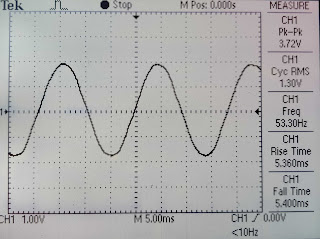270 Kv Odrive D5065

All of these motors are 12N14P and of a similar construction. Its clear that the shape of the back EMF is sinusoidal and not trapezoidal. Therefore, these motors are technically permanent magnet synchronous motors (PMSM) and not brushless DC motorsOverall, the measured K_{V} of each motor was quite close to their labelled values as will be shown in a table later.

To estimate K_{\tau} the output torque of each motor was measured with respect to the armature current. This was done by winding a string around the rotor of each motor, attaching that string to a lever arm that then pulled down onto a laboratory balance. This balance then measured the weight, and therefore force, produced by the motor. This method is only possible thanks to the use of a high resolution encoder (8192 counts per rotation) and an Odrive motor controller which uses field oriented control (FOC) to place all current on the motors q-axis for maximum torque, even when stationary.

The setup is crude and would have been much cleaner if I had just attach an arm to each motors rotor. However, by using a string I didn't need to make a new arm adaptor for each motor and could instead just consider the diameter of the rotor in the final calculations. This was enough to get the job done.

Using this setup and a simple script I slowly stepped up the commanded current for each motor  while manually recording the weight on the scale. After some back calculations the torque output for each motor with commanded current was found.

No surprises so far with the bigger motors producing more torque per amp and the torque increasing linearly with current.

A summary of the motor parameters can be seen below and the raw data can be found here

Racerstar BR2212 Turnigy SK3 5055 Odrive D5065 Keda 6364 Odrive D6374
Rated kV rpm/V 1000 280 270 190 150
Measured kV rpm/V 1058 276 259 182 151
Phase Resistance Ohm 0.128 0.032 0.039 0.039 0.039
Phase Inductance H 1.84E-05 1.33E-05 2.02E-05 2.13E-05 2.81E-05
Weight kg 0.045 0.389 0.411 0.647 0.885
Price $USD 6 52 69 47 99 Torque constant (Kt) N·m/A 0.008 0.029 0.030 0.042 0.053 Kt/kg N·m/A/kg 0.172 0.073 0.073 0.065 0.060 Kt/$ USD N·m/A/\$ USD 0.00129 0.00055 0.00043 0.00090 0.00054
Motor constant (Km) N⋅m/sqrt(W) 0.02 0.16 0.15 0.21 0.27
Km/kg N⋅m/sqrt(W)/kg 0.485 0.417 0.369 0.329 0.308
Conversion constant 8.1 8.2 8.2 8.2 8.2

I have calculated the 'conversion constant' based on an average of a few different current vs torque measurements. The calculated 'conversion constant'  is actually very close to the theoretical value, with measured values between 8.1 and 8.2. Any error is likely due to the friction in the system and my less than ideal measurement setup. Also note that these values are based on the manufacture rated K_{V} of the motors and they are little lower if my own K_{V} is used instead. I'm not sure why this is the case but it may have something to do with the 'fudge factor' each manufacture assumes when estimating K_{V}.

Also listed is K_{\tau} with respect to motor weight and motor cost. Surprisingly, the smallest motor (1000 K_{V} BR2212) came out on top by a considerable margin in both cases, with a K_{\tau} more than double that of the other motors. This suggests that the electrical loading (current density in the copper windings) is much higher in the smallest motor when compared to the others. This same result could be achieved for the other motors by re-winding them to have a lower K_{V}. However, since the 'torque efficiency' (torque produced per Watt) is the same no matter how a motor is wound provided the amount of copper remains the same, and that the mass fraction of copper is likely to be about the same for these motors, the smallest motor will produce no more torque per unit weight than the rest when thermally limited. This assumption is backed up by the fact that the motor constant (K_{M}) per weight is about the same for all motors tested. Its likely that the motor manufacture decided to wind the smallest motor this way so that its base speed matched that required by an appropriately sized prop and the typical battery voltage used on model aircraft and drones.

## Limitations of this approach

Using K_{V} and a motors current draw to estimate its torque output only works provided that:
• A motor does not produce any useful reluctance torque (i.e. its a  non-salient machine**). This is true for essentially all 'hobby grade' electric motors.
• A motors torque increase linearly with current. This is not true if your motor is close to saturation. However, most 'hobby grade' electric motors are designed to operate with a current limit well below that needed to saturate them and so this is generally a safe assumption for stead state use.
• A motor is operated below its base speed. Operating a motor above its base speed by field weakening effectively lowers a motors K_{V} in that region and so unless you know by how much K_{V} is reduced you can not calculate the torque output. However, since no 'hobby grade' motor controllers (ESC's) that I know of utilise field weakening this is also not an issue.
• The current waveform supplied by a motor controller exactly matches a motors back EMF waveform (i.e. the current is not exactly on the q-axis at all times). This is generally true when using field oriented control (FOC) on a PMSM motor that has inbuilt position sensors (hall effect, encoder etc.). Operating a motor with 'six step 120 degree' commutation at low speed without a position sensor will result in less torque being produced than that predicted using the 'conversion constant' while high speed operation should be pretty close.

## Conclusion

The torque produced by brushless permanent magnet synchronous motor can be easily estimated so long as its K_{V} and armature current is known. This relationship works because a motors K_{V} is fundamentally the same thing as its motor torque constant provided the right units are used, which is where a 'conversion constant' of ~8.3 is required.

* The armature is considered the winding in which a rotational 'back emf' would be generated if the motor were used as a generator. In some motor designs the armature is on the rotor (e.g. brushed DC motor) or in the stator (e.g. brushless DC motor).

** A non-salient machine in this context is any motor which does not derive useful torque from reluctance torque. A motor can have salient poles on the rotor or stator and still be considered a non-salient machine with this definition.

Equations were produced in this post with the help of arachnoid.com. If you have noticed any errors in the above article then please let me know.

## Sunday, December 23, 2018

### Why most hobby grade BLDC out runners are actually permanent magnet synchronous motors (PMSM)

Look anywhere online for a brushless direct current (BLDC) electric motor and you will likely find something that looks like the out-runner shown below: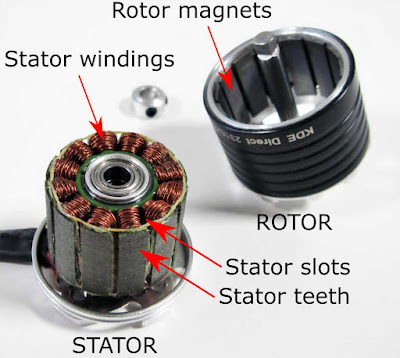A hobby grade 'BLDC' motor
However, it turns out this is technically not a BLDC motor. By most definitions, it is actually a permanent magnet synchronous motor (PMSM). If you own a similar looking out-runner style hobby motor then you too may have a PMSM.

This post will briefly cover the difference between a BLDC motor and a PMSM and when this difference matters.

## BLDC vs PMSM

BLDC and PMSM have a lot in common. Neither have brushes, both have permanent magnets on the rotor and have an armature on the stator. Where they differ is in how the magnetic field produced by the rotor magnets interacts with the windings of the armature.

Let's consider an 'out-runner' motor of the style shown below.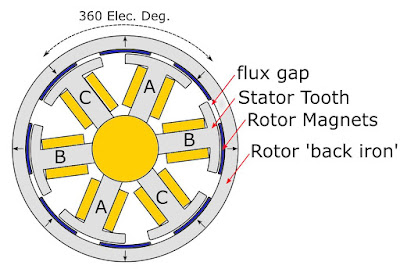A cross-sectional view of a 6 slot, 8 pole (6N8P) motor
The movement of the rotor magnets past the stator teeth induces a back EMF (bEMF) into the windings of the armature. When the phase-phase bEMF is plotted with electrical angle (360 electrical degrees = 360 mechanical degrees / no. pole pairs) it may look sinusoidal, trapezoidal, or somewhere in between.

If a motor produces a trapezoidal bEMF then it is considered BLDC motor since this shape is similar to what would be seen from a conventional brushed DC motor. If your motor produces a sinusoidal bEMF then it is generally considered to be a PMSM.

## How to tell if your motor is a BLDC or PMSM? Measure its bEMF

If you have access to an oscilloscope then determining if your motor is a PMSM or a BLDC motor is as simple as measuring across any two phases and spinning the rotor to observe the bEMF shape. This can be done by hand for low kV motors or with the help of a power drill for high kV motors. After doing this for a 12 slot, 14 pole (12N14P) out-runner the following shape was seen:Back EMF produced by a 12N14P out-runnerD5065 270 kV 12N14P brushless out-runner from Odrive Robotics
This motor clearly has a sinusoidal bEMF and so would be considered a PMSM. Repeating this same measurement for a couple of other motors from different manufacturers also yielded the same results.

A sinusoidal bEMF typically means a motor has been wound with distributed windings, where the windings are distributed over many slots, and is more common for large electric motors. Distributed windings are easily spotted by the overlap at the end of the motor.

However, hobby grade brushless motors are almost universally constructed with concentrated windings. It is, therefore, a little surprising that they can produce a sinusoidal bEMF. The reason for a sinusoidal bEMF in the out-runner tested above is apparently related to its 12N14P configuration in combination with its doubly wound concentrated windings. I will explore this in more detail at some point in the future. Note that so far I have only measured 12N14P out-runner style motors and so you may see different results for motors with different slot/pole ratios or for in-runners.

## BLDC or PMSM - Does it matter?

Useful torque is produced by an electric motor when you feed in a current waveform to each phase that perfectly opposes the a generated bEMF. Importantly, the shape of the waveform does not matter, so long as it exactly matches the bEMF waveform. For example, consider the torque produced by a PMSM and BLDC motor as seen by the figure below which were taken from James Mavey's excellent masters thesis.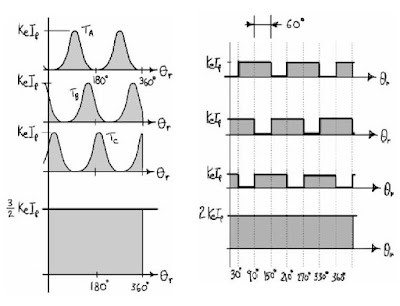Torque output vs current input for a PMSM (left) and a BLDC (right) motor
When you sum up the current contributions from each phase for the sinusoidal waveform (PMSM) and for the trapezoidal waveform (BLDC) you see the same result; a perfect constant output current, and therefore a constant output torque. Also, in theory at least, both motors should be equally efficient. In reality, even if you could perfectly match the current to the bEMF, the rapid change in flux density seen by the stator in a BLDC motor due to the use of a trapezoidal waveform is likely to induce larger eddy current losses than a comparable sinusoidal PMSM.

The problems begin when you use a motor controller that outputs a current waveform which does not exactly match the bEMF of your motor. Most low-cost hobby grade motor controllers (ESC's) only output a 'six-step 120 degree' current waveform like that shown for the BLDC motor above. Therefore, if you use a PMSM with one of these ESC's it's torque output will be choppy, which creates audible noise, vibration, and will be quite inefficient. Instead, you would ideally use a motor controller which supports field oriented controlled (FOC) and that outputs a sinusoidal current waveform that more closely matches that of your motor.

Furthermore, the trapezoidal bEMF produced by a BLDC motor can vary quite a lot from motor to motor. This means that the current waveform produced by an ESC will never perfectly match the bEMF of a BLDC motor. This means that even if you match a BLDC motor with an ESC you will still have some amount of motor noise, vibration, and decreased efficiency. A PMSM has no such problem since ideally, ever motor produces the same sinusoidal bEMF.

For most hobby applications (e.g. small model planes, boats, and cars) using a PMSM with a conventional six step ESC won't cause any noticeable problems. However, for high-performance applications (e.g. multi-rotors used for cinematography, robotics and EV applications) the reduced noise, vibration and increased efficiency that comes from using a FOC motor controller with a PMSM may mean it's worth the extra investment.

## Conclusion

If a motor produces a sinusoidal bEMF then its a PMSM and not a BLDC motor. A PMSM is best driven by a sinusoidal current as this reduces noise, vibration and improves efficiency.

In order to avoid confusion, going forward I will be simply referring to both BLDC and PMSM as brushless permanent magnet synchronous motors (PMSM).

If you have noticed any errors in the above article then please let me know.